# GPoM : Models predictability

## Model skills

To evaluate a model skills is a difficult problem when the studied dynamic is characterized by a high sensitivity to the initial conditions, which is the case of many real world system (climatic, hydrological, epidemiological, ecological systems, etc.). Indeed, such high sensitivity has the following consequence: a small difference in the initial state of the system (real or modeled) can lead to different trajectories, even for the same governing equations. The consequence of this situation is that, for slightly different initial conditions, the data set used to validate (but also to estimate or to optimize) the model may have been very different.

One solution to avoid this difficulty is to use nonlinear invariants to validate the model. Nonlinear invariants are independent to the initial conditions and are thus well adapted in their design for this purpose. Three types of nonlinear invariants can be used: dynamical1, geometrical2 and topological3. In their principle, these invariants may provide a interesting measures for the validation, the calibration etc. Unfortunately, in practice, these may also have important limitations. One difficulty met with dynamical and geometrical invariants is that theit algorithms are generally quite sensitive to noise and thus difficult to use for a robust validation when datasets from real world conditions are considered. Topological invariants are less sensitive to noise but their application is mainly limited to three-dimensional systems, although attempts have been made to extend their use to higher dimensions4, 5, 6.

Alternative approaches may thus be preferred, especially when considering real world situations and noisy time series. Several alternatives of validations are given in reference 7. Model predictability is one of these alternatives and is based on analyzing the forecast error growth8, 9.

## Predictability

One practical way to analyze a model skills is to quantify its forecasting error growth. To do so, it is necessary to launch the forecasts from some konwn initial conditions and to compare this forecast to the original time series.

To illustrate the method, the global modelling technique is first applied in order to get an ensemble of models for which predictability will be tested.

  # load data
data("Ross76")
# time vector
tin <- Ross76[seq(1, 3000, by = 8), 1]
# single time series, here y(t) is chosen
data <- Ross76[seq(1, 3000, by = 8), 3]
# global modelling
# results are put in list outputGPoM
outputGPoM <- gPoMo(data[1:300], tin = tin[1:300], dMax = 2, nS=c(3),
show = 0, method = 'rk4',
nPmax = 12, IstepMin = 400, IstepMax = 401)
## ### For Istep = 400 (max: 401), models to test: 10 / 10
## ### Number of unclassified models: 8 / 10

Eight models are here obtained:

  sum(outputGPoM$okMod) ##  8  which(outputGPoM$okMod == 1)
##   1  2  3  5  6  7  9 10

For one single initial condition, the forecasting error is defined as:

$$e(t,h) = y(t+h) - y^{obs}(t+h)$$.

Such a forecasting error $$e$$ can be estimated for one model, starting from one chosen initial state. Model #1 is defined by the following set of equations:

  visuEq(3, 2, outputGPoM$models$model1)
## dX1/dt = X2
##
## dX2/dt = X3
##
## dX3/dt = 0.516427583533666 X1 X2

The initial state can be chosen as:

  x0 <- head(outputGPoM$filtdata, 1)[1:3] This model can be integrated numerically, starting from this initial condition x0 using the numicano function, and providing the prediction $$y(t)$$ to be compared to the original time series $$y^{obs}(t)$$.  ############### # forecasting # ############### outNumi <- numicano(nVar = 3, dMax = 2, Istep = 100, onestep = 0.08, KL = outputGPoM$models$model7, v0 = x0, method = 'rk4') plot(outputGPoM$tfilt, outputGPoM$filtdata[,1], xlim = c(0,10), type='l', main = 'Observed and simulated', xlab = expression(italic(t)), ylab = expression(italic(y(t)))) lines(outNumi$reconstr[,1], outNumi$reconstr[,2], type='l', col = 'red') nbpt <- length(outNumi$reconstr[,2])
lines(c(-5,30), c(0,0), type='l', col = 'gray')
lines(outNumi$reconstr[,1], outNumi$reconstr[,2] - outputGPoM$filtdata[1:nbpt,1], type='l', col = 'green')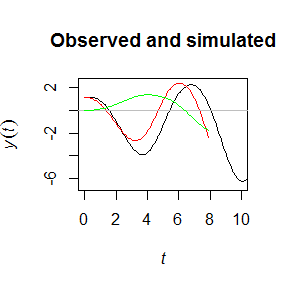Since the system is characterized by a high sensitivity to the initial conditions, forecasting error growth may depend on the chosen initial states. To get a more general picture of the error growth (here plotted in green for the chosen initial condition x0), such error should be reestimated several times starting from other initial states which is the aim of the predictab function. With this function, predictability can be applied automatically to these eight models, for an ensemble of Nech initial conditions, and on prediction horizons of hp steps corresponding here to a time interval of 1.2 (since time sampling of tin equals 0.08, see upper):  ####################### # test predictability # ####################### outpred <- predictab(outputGPoM, hp = 15, Nech = 30, selecmod = 9, show = 0)  # manual visualisation of the outputs (e.g. for model 9): plot(c(outpred$hpE, max(outpred$hpE)), c(0,0), type='l', main = 'Error growth', xlab = expression(h), ylab = expression(italic(e(h))), ylim = c(min(outpred$Errmod9), max(outpred$Errmod9))) for (i in 1:dim(outpred$Errmod9)) {
lines(outpred$hpE, outpred$Errmod9[,i],
col = 'green')
}
lines(c(outpred$hpE, max(outpred$hpE)), c(0,0), type='l')
# in terme of variance
# manual visualisation of the outputs (e.g. for model 9):
plot(c(outpred$hpE, max(outpred$hpE)), c(0,0),
type='l', main = 'Square error growth',
xlab = expression(italic(h)), ylab = expression(italic(e^2) (italic(h))),
ylim = c(0, 0.25*max(outpred$Errmod9)^2)) for (i in 1:dim(outpred$Errmod9)) {
lines(outpred$hpE, outpred$Errmod9[,i]^2,
col = 'green')
}
lines(c(outpred$hpE, max(outpred$hpE)), c(0,0), type='l')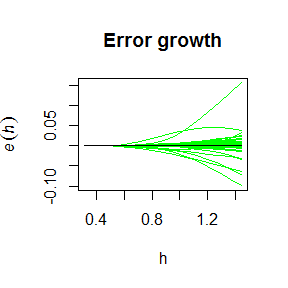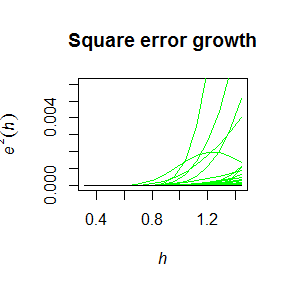Function predictab can be applied to several models and the forecasted error growth $$e(t,h)$$ can also be plotted as a bidimensionnal representation, where the color represents the forecasting error (note that different palettes are here used for the two plots):

  #######################
# test predictability #
#######################
outpred <- predictab(outputGPoM, hp = 15, Nech = 30,
selecmod = c(1,9), show = 0)
  # manual visualisation of the outputs (e.g. for model 1):
image(outpred$tE, outpred$hpE, t(outpred$Errmod1), xlab = expression(italic(t)), ylab = expression(italic(h)), main = expression(italic(e[model1](t,h)))) # (e.g. for model 9): image(outpred$tE, outpred$hpE, t(outpred$Errmod9),
xlab = expression(italic(t)), ylab = expression(italic(h)),
main = expression(italic(e[model9])(italic(t),italic(h))))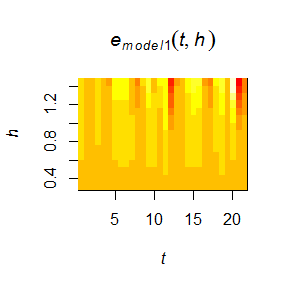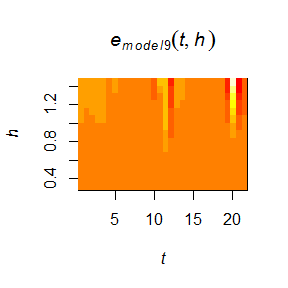To have an interpretation of this kind of plot, see 10. By default, the predictab function will be applied to all the unclassified models (that is such as okMod == 1). An overview can also be obtained at a glance using the default parameterization that is such as show = 1.

  #######################
# test predictability #
#######################
outpred <- predictab(outputGPoM, hp = 15, Nech = 30)

## Epilogue

You have reached the end of this tutorial. You should be able now to use most of the tools provided in the GPoM package. We hope you can enjoy these package when applying it to your favorite data sets. When presenting the results, please kindly quote the appropriate references to refer to the package, and to the various techniques used (see the introducing vignette GeneralIntro).

1. A. Wolf, J. B. Swift, H. L. Swinney, & J. A. Vastano, 1985. Determining Lyapunov exponents from a time series, Physica D 16, 285-317.

2. P. Grassberger and I. Procaccia, 1983. Characterization of strange attractors, Phys. Rev. Lett., 50, 346-349.

3. R. Gilmore, 1998. Topological analysis of chaotic dynamical systems, Rev. Mod. Phys., 70, 1455-1530.

4. R. Gilmore & M. Lefranc, The Topology of Chaos (Wiley, New York), 2002.

5. S. Mangiarotti, Modélisation globale et caractérisation topologique de dynamiques environnementales: de l’analyse des enveloppes fluides et du couvert de surface de la Terre à la caractérisation topolodynamique du chaos, dissertation Habilitation to Direct Researches, Université de Toulouse, 2014.

6. S. Mangiarotti & C. Letellier, 2015. Topological analysis for designing a suspension of the Hénon map, Phys. Lett. A, 379, 3069-3074.

7. S. Mangiarotti, M. Peyre & M. Huc, 2016. A chaotic model for the epidemic of Ebola virus disease in West Africa (2013–2016). Chaos, 26, 113112.

8. S. Mangiarotti, L. Drapeau & C. Letellier, 2014. Two chaotic global models for cereal crops cycles observed from satellite in Northern Morocco, Chaos, 24, 023130.

9. S. Mangiarotti, 2015. Low dimensional chaotic models for the plague epidemic in Bombay (1896-1911), Chaos, Solitons & Fractals, 81(A), 184-196.

10. S. Mangiarotti, P. Mazzega, P. Hiernaux, and E. Mougin, 2012. Predictability of vegetation cycles over the semi-arid region of Gourma (Mali) from forecasts of AVHRR-NDVI signals, Remote Sens. Environ., 123, 246-257.